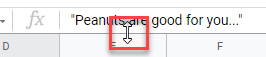# What is the Formula Bar in Excel & Google Sheets?

This tutorial explains the function of the formula bar in Excel and Google Sheets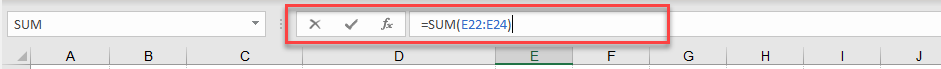The formula bar in Excel is above the column headers and below the Ribbon. The bar has the name box on the left, and the formula bar on the right.

## Hide and Unhide the Formula Bar

If you cannot see the formula bar, it was probably hidden. There is an option in the Ribbon to show or hide the formula bar.

In the Ribbon, go to View > Show > Formula Bar.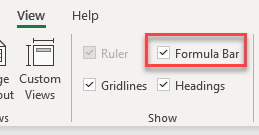## Purpose of the Formula Bar

When you select a cell in a worksheet, the value in that cell shown in the formula bar either as a value/text or as a formula. You can use the formula bar to edit cells instead of typing directly into a cell.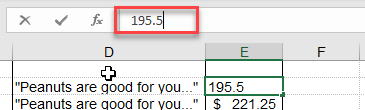If you have formatted the data in your active cell (e.g., as a currency), then your worksheet displays the value in the cell as formatted. The formula bar, on the other hand, only shows the data in its “raw” data format without any formatting applied.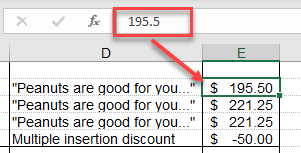Similarly, if your cell contains a formula, the formula bar displays that formula, and the active cell displays the result.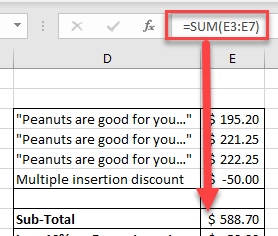If a number has been formatted to have two decimal places but actually has a lot more (e.g., an interest rate), the formula bar shows all the decimal places, while the worksheet shows the number formatted to two decimal places.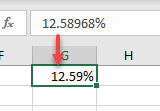## View and Create Formulas

One of the most fundamental purposes of the formula bar is the ability to view and create formulas.

If you click on the cell that contains the formula, the formula shows up in the formula bar, while the result is shown in the active cell in the worksheet itself.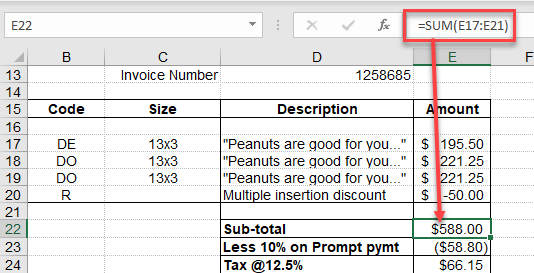To create a formula, either type it into the cell, or enter directly into the formula bar.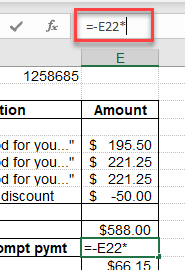## Expand the Formula Bar

If you have a particularly long formula, you can expand the formula bar either horizontally or vertically.

1. To expand the formula bar vertically, move your mouse to the bottom of your formula bar until the cursor changes to a double-headed arrow.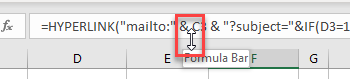1. Click and drag the bottom of the formula bar down to increase its size.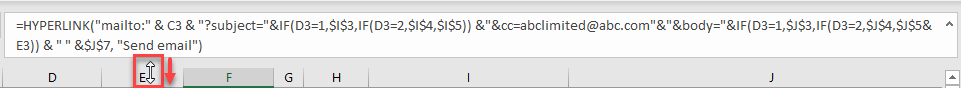If a single cell contains multiple lines of text, you can drag the formula bar down to show all the text in the cell.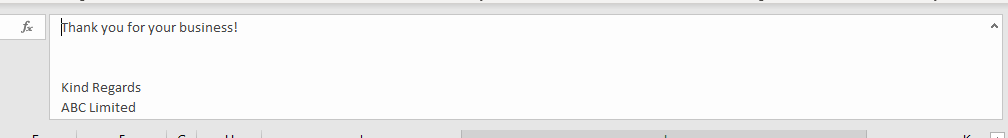1. To expand the formula bar horizontally, move your mouse cursor between the name box and the formula bar. It changes to a double-headed arrow.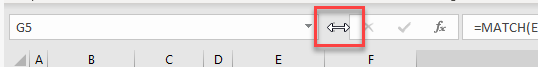1. Click and drag to the left to adjust the horizontal size of your formula bar.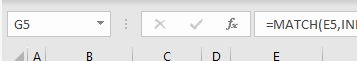You can also use the keyboard shortcut CTRL + SHIFT + U to expand and contract the formula bar. This adjusts it to a standard size or put it back to the default size.

## What is the Formula Bar in Google Sheets?

The formula bar in Google Sheets works very similarly.

As in Excel, the formula bar sits in the Google Sheet below the Menu and above the column headers.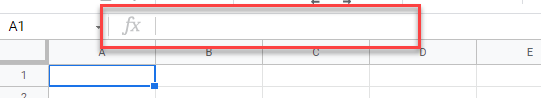You can view the raw data in the formula bar and the formatted data in the active cell in the sheet.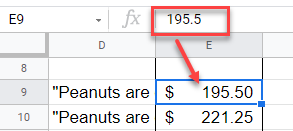Or view the formula in the formula bar while seeing the result of that formula in the sheet.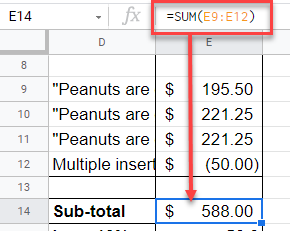You can edit the text or formula in the formula bar as well as directly in the sheet, and as in Excel, you can expand and contract the formula bar by placing your mouse at the bottom of the formula bar so your mouse pointer changes to a double-headed arrow, and dragging down until the formula bar is the right size.